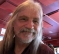## Low-pass filter image dataStarted by 3 years ago11 replieslatest reply 3 years ago223 views

I have some image data that I want to smooth out, but I want it to smooth out nicely to the edges.

Fourier transforms wouldn't do this without windowing -- if you tried, you'd just weird effects where the left edge would bleed into the right, and the top into the bottom.

Are there any known techniques for smoothing a whole image that don't get tripped up by the edges?

[ - ]There is no information from outside the image. Thus a smoother  cannot guess that information to operate perfectly along the edges. (Same holds for filtering 1D signals.) Standard approaches are to use cyclic/mirror boundary cconditions to preserve continuity along the edges. If using DFT/FFT, simply extend the image with zeroes symmetrically (usually for DFT double the size of the input image), the circular convolution and "perfect" interpolation properties of the DFT will take care of the rest. Then extract the central part of the output image of the size of the input image.

For filtering in the image/"time" domain directly (convolution or nonlinear filters such as median, erosion, dilation) extend the image with zeroes, mirror images (basically same as DFT approach above) or repetition of the edge pixels: you'll have different results and choose what you like most or what your application can use best. There is no single "correct" way of doing it.

[ - ]i really dunno diddley, Tim, but isn't the DCT (there are four or five forms) essentially a DFT of 4 times the size (twice in X and twice in Y) where the added image is a mirror image of the original?  so there is continuity in the zeroth derivative.

i thought that was to deal with edges and periodicity.

or is it the LPF filtering that mushes up the edges?

[ - ]I've been going off of this.  Which is nice, but isn't long on expositions on how to use it: http://epubs.siam.org/doi/pdf/10.1137/S00361445983...

[ - ]On a different note I just noticed that DCT was invented by a fellow called Nasir Ahmed in 1972 and should have been named after him to be fair.

Just like Costas loop, Thevenin equivalent, Hilbert transform, Fourier Transform, Farrow filter, Goertzel algorithm, Zadoff-Chu sequence and the list goes on.

[ - ]Another post I need to make is good books that include information on the DCT!  Something that's not over-the-top mathematically, yet is still rigorous enough that I can figure out when to use what type.

I'm actually experimenting with using the DCT for this, with good results:

• Take DCT of the image
• Multiply the upper left corner of the DCT with 0.5 * (1 + cos(n/N * pi)) for N points followed by zeros
• Take IDCT of the result

It's not exactly speedy, but it's going to be used in an off-line calibration operation for an optical instrument (I want to separate the effects of pixel-by-pixel processes from those of processes that essentially "tilt and wiggle" the image across the sensor).

I ran across an interesting paper on the DCT that starts by saying that the FFT is what you get by assuming sinusoids and repeating boundary conditions (i.e., x[n + N] = x[n]), while the various forms of the DCT assume either the Dirchlet boundary condition or the -- um -- other one (I gotta brush up on my math).  So for the "jpg DCT" x[-1] = x[N] = 0 (Dirchlet).

[ - ]does this work or did dsprelated just eat my reply to Tim?
[ - ]It seems to work, and if there's some other reply from you, it's gone.

I'm looking for more info because I dislike having something that works dandy in practice but I don't have a theoretical basis for.

(Which is better than having something that works in theory but not in practice -- but still.)

[ - ]this is what i meant to say, but when i hit "Submit Reply", the response was something like "You must be logged in to reply" and it totally lost about 5 or 6 paragraphs.

as best as i can tell (just from reading Wikipedia), the DCT (in one dimension) takes this finite set of data of N samples:

x, x, x, ... x[N-2], x[N-1]

and it extends it to

x, x, ... x[N-2], x[N-1], x[N-1], x[N-2], ... x, x

and runs a length 2N DFT on that or it extends it as

x, x, ... x[N-2], x[N-1], x[N-2], x[N-3], ... x, x

and runs a length 2N-2 DFT on that data and in both cases removes the redundancy (due to even symmetry) in the X[k] domain.  in the former case, the input sequence is symmetrical about a line 1/2 sample to the left and that offset and linear phase shift is removed in the DCT.  in the latter case, it is already symmetrical about x, there is no offset, and only the cosine terms exist.

in both cases the data is made continuous in the zeroth derivative.

this time i am selecting all, and copying text, before hitting Submit Reply.

[ - ]Forgive my ignorance but is there any trick that can be done with interpolation that would create extra data (n-1 zeroes) at the edges and in between each actual sample and then maybe you could decimate afterwards?

[ - ]Here is a super simple way to get the smoothing you want.  Just smooth the pixels from left to right with an EMA.  Then take that result and smooth it from right to left with the same EMA.  You will get double smoothing and the lags are cancelling, leaving the edges intact.  Then smooth from top to bottom with the same EMA.  Finally, smooth that result from bottom to top with the same EMA.  Of course, you will have to format your pixels into rows and columns.

[ - ]